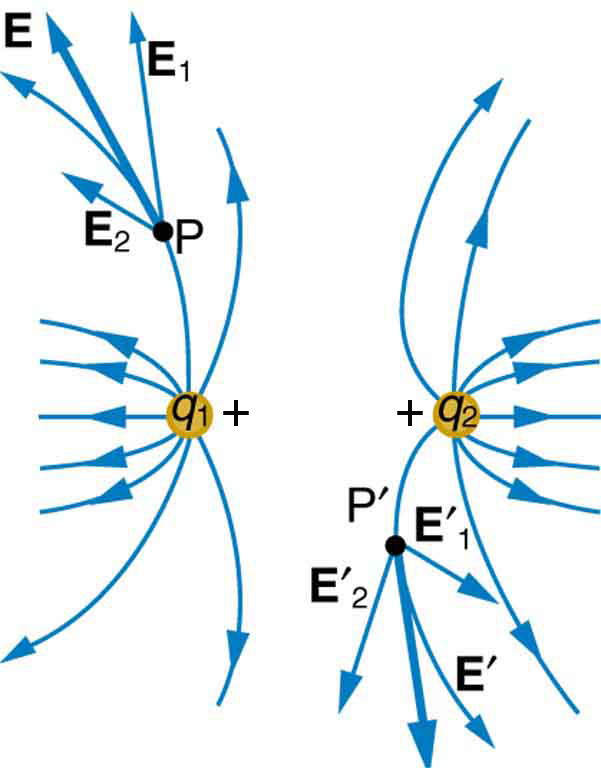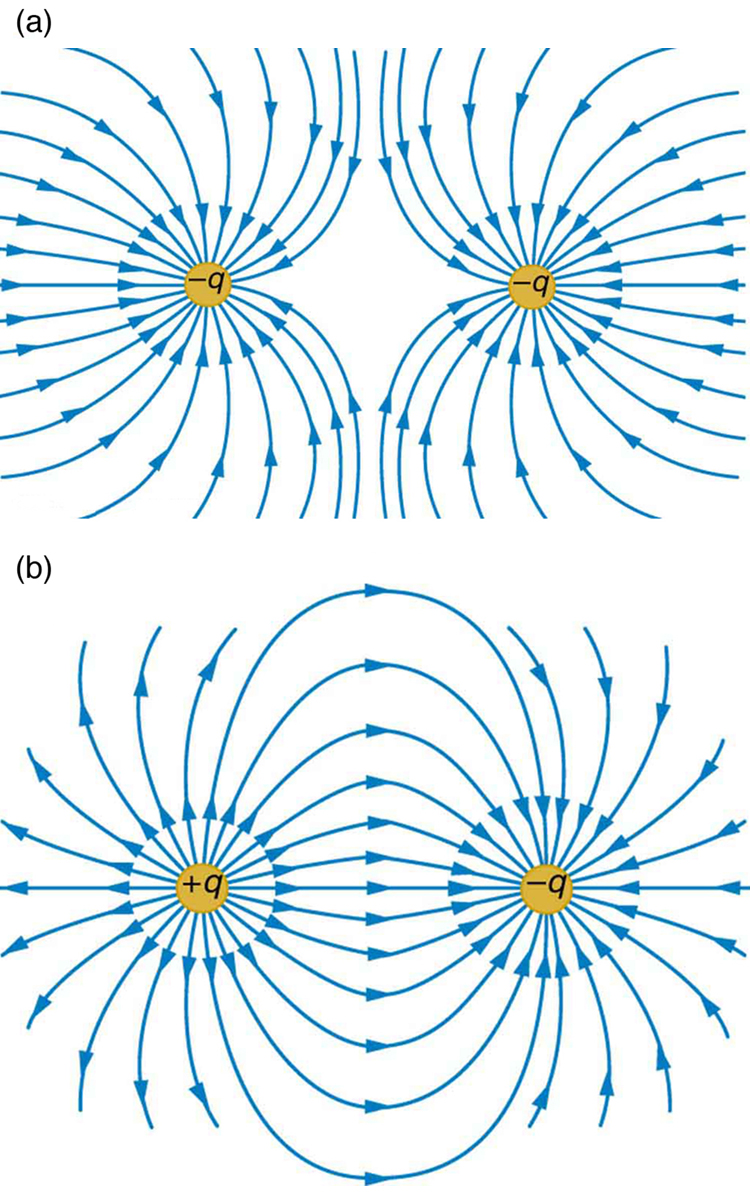# 18.5 Electric field lines: multiple charges  (Page 2/7)

 Page 2 / 7

Solution

The electric field strength at the origin due to ${q}_{1}$ is labeled ${E}_{1}$ and is calculated:

$\begin{array}{}{E}_{1}=k\frac{{q}_{1}}{{r}_{1}^{2}}=\left(8\text{.}\text{99}×{\text{10}}^{9}\phantom{\rule{0.25em}{0ex}}\text{N}\cdot {\text{m}}^{2}{\text{/C}}^{2}\right)\frac{\left(5\text{.}\text{00}×{\text{10}}^{-9}\phantom{\rule{0.25em}{0ex}}\text{C}\right)}{{\left(2\text{.}\text{00}×{\text{10}}^{-2}\phantom{\rule{0.25em}{0ex}}\text{m}\right)}^{2}}\\ {E}_{1}=1\text{.}\text{124}×{\text{10}}^{5}\phantom{\rule{0.25em}{0ex}}\text{N/C}.\end{array}$

Similarly, ${E}_{2}$ is

$\begin{array}{}{E}_{2}=k\frac{{q}_{2}}{{r}_{2}^{2}}=\left(8\text{.}\text{99}×{\text{10}}^{9}\phantom{\rule{0.25em}{0ex}}\text{N}\cdot {\text{m}}^{2}{\text{/C}}^{2}\right)\frac{\left(\text{10}\text{.}0×{\text{10}}^{-9}\phantom{\rule{0.25em}{0ex}}\text{C}\right)}{{\left(4\text{.}\text{00}×{\text{10}}^{-2}\phantom{\rule{0.25em}{0ex}}\text{m}\right)}^{2}}\\ {E}_{2}=0\text{.}\text{5619}×{\text{10}}^{5}\phantom{\rule{0.25em}{0ex}}\text{N/C}.\end{array}$

Four digits have been retained in this solution to illustrate that ${E}_{1}$ is exactly twice the magnitude of ${E}_{2}$ . Now arrows are drawn to represent the magnitudes and directions of ${\mathbf{\text{E}}}_{1}$ and ${\mathbf{\text{E}}}_{2}$ . (See [link] .) The direction of the electric field is that of the force on a positive charge so both arrows point directly away from the positive charges that create them. The arrow for ${\mathbf{\text{E}}}_{1}$ is exactly twice the length of that for ${\mathbf{\text{E}}}_{2}$ . The arrows form a right triangle in this case and can be added using the Pythagorean theorem. The magnitude of the total field ${E}_{\text{tot}}$ is

$\begin{array}{lll}{E}_{\text{tot}}& =& \left({E}_{1}^{2}+{E}_{2}^{2}{\right)}^{\text{1/2}}\\ & =& {\left\{\left(\text{1.124}×{\text{10}}^{5}\phantom{\rule{0.25em}{0ex}}\text{N/C}{\right)}^{2}+\left(\text{0.5619}×{\text{10}}^{5}\phantom{\rule{0.25em}{0ex}}\text{N/C}{\right)}^{2}\right\}}^{\text{1/2}}\\ & =& \text{1.26}×{\text{10}}^{5}\phantom{\rule{0.25em}{0ex}}\text{N/C.}\end{array}$

The direction is

$\begin{array}{lll}\theta & =& {\text{tan}}^{-1}\left(\frac{{E}_{1}}{{E}_{2}}\right)\\ & =& {\text{tan}}^{-1}\left(\frac{1\text{.}\text{124}×{\text{10}}^{5}\phantom{\rule{0.25em}{0ex}}\text{N/C}}{0\text{.}\text{5619}×{\text{10}}^{5}\phantom{\rule{0.25em}{0ex}}\text{N/C}}\right)\\ & =& \text{63}\text{.}4º,\end{array}$

or $63.4º$ above the x -axis.

Discussion

In cases where the electric field vectors to be added are not perpendicular, vector components or graphical techniques can be used. The total electric field found in this example is the total electric field at only one point in space. To find the total electric field due to these two charges over an entire region, the same technique must be repeated for each point in the region. This impossibly lengthy task (there are an infinite number of points in space) can be avoided by calculating the total field at representative points and using some of the unifying features noted next.

[link] shows how the electric field from two point charges can be drawn by finding the total field at representative points and drawing electric field lines consistent with those points. While the electric fields from multiple charges are more complex than those of single charges, some simple features are easily noticed.

For example, the field is weaker between like charges, as shown by the lines being farther apart in that region. (This is because the fields from each charge exert opposing forces on any charge placed between them.) (See [link] and [link] (a).) Furthermore, at a great distance from two like charges, the field becomes identical to the field from a single, larger charge.

[link] (b) shows the electric field of two unlike charges. The field is stronger between the charges. In that region, the fields from each charge are in the same direction, and so their strengths add. The field of two unlike charges is weak at large distances, because the fields of the individual charges are in opposite directions and so their strengths subtract. At very large distances, the field of two unlike charges looks like that of a smaller single charge.Two positive point charges q 1 size 12{q rSub { size 8{1} } } {} and q 2 size 12{q rSub { size 8{2} } } {} produce the resultant electric field shown. The field is calculated at representative points and then smooth field lines drawn following the rules outlined in the text.(a) Two negative charges produce the fields shown. It is very similar to the field produced by two positive charges, except that the directions are reversed. The field is clearly weaker between the charges. The individual forces on a test charge in that region are in opposite directions. (b) Two opposite charges produce the field shown, which is stronger in the region between the charges.

how do you calculate the 5% uncertainty of 4cm?
4cm/100×5= 0.2cm
haider
how do you calculate the 5% absolute uncertainty of a 200g mass?
= 200g±(5%)10g
haider
use the 10g as the uncertainty?
melia
haider
topic of question?
haider
the relationship between the applied force and the deflection
melia
sorry wrong question i meant the 5% uncertainty of 4cm?
melia
its 0.2 cm or 2mm
haider
thank you
melia
Hello group...
Chioma
hi
haider
well hello there
sean
hi
Noks
the meaning of phrase in physics
is the meaning of phrase in physics
Chovwe
write an expression for a plane progressive wave moving from left to right along x axis and having amplitude 0.02m, frequency of 650Hz and speed if 680ms-¹
how does a model differ from a theory
To use the vocabulary of model theory and meta-logic, a theory is a set of sentences which can be derived from a formal model using some rule of inference (usually just modus ponens). So, for example, Number Theory is the set of sentences true about numbers. But the model is a structure together wit
Jesilda
with an iterpretation.
Jesilda
what is vector quantity
Vector quality have both direction and magnitude, such as Force, displacement, acceleration and etc.
Besmellah
Is the force attractive or repulsive between the hot and neutral lines hung from power poles? Why?
what's electromagnetic induction
electromagnetic induction is a process in which conductor is put in a particular position and magnetic field keeps varying.
Lukman
wow great
Salaudeen
what is mutual induction?
je
mutual induction can be define as the current flowing in one coil that induces a voltage in an adjacent coil.
Johnson
how to undergo polarization
show that a particle moving under the influence of an attractive force mu/y³ towards the axis x. show that if it be projected from the point (0,k) with the component velocities U and V parallel to the axis of x and y, it will not strike the axis of x unless u>v²k² and distance uk²/√u-vk as origin
show that a particle moving under the influence of an attractive force mu/y^3 towards the axis x. show that if it be projected from the point (0,k) with the component velocities U and V parallel to the axis of x and y, it will not strike the axis of x unless u>v^2k^2 and distance uk^2/√u-k as origin
No idea.... Are you even sure this question exist?
Mavis
I can't even understand the question
yes it was an assignment question "^"represent raise to power pls
Gabriel
Gabriel
An engineer builds two simple pendula. Both are suspended from small wires secured to the ceiling of a room. Each pendulum hovers 2 cm above the floor. Pendulum 1 has a bob with a mass of 10kg . Pendulum 2 has a bob with a mass of 100 kg . Describe how the motion of the pendula will differ if the bobs are both displaced by 12º .
no ideas
Augstine
if u at an angle of 12 degrees their period will be same so as their velocity, that means they both move simultaneously since both both hovers at same length meaning they have the same length
Modern cars are made of materials that make them collapsible upon collision. Explain using physics concept (Force and impulse), how these car designs help with the safety of passengers.
calculate the force due to surface tension required to support a column liquid in a capillary tube 5mm. If the capillary tube is dipped into a beaker of water
find the time required for a train Half a Kilometre long to cross a bridge almost kilometre long racing at 100km/h
method of polarization
Ajayi
What is atomic number?
The number of protons in the nucleus of an atom
Deborah

#### Get Jobilize Job Search Mobile App in your pocket Now!ByByBy Robert MurphyBy OpenStaxByBy Janet ForresterBy Marion CabalfinBy OpenStaxBy OpenStaxByBy Kimberly NicholsBy Stephen Voron